×#### Thank you for registering.

One of our academic counsellors will contact you within 1 working day.

Click to Chat

1800-1023-196

+91-120-4616500

CART 0

• 0

MY CART (5)

Use Coupon: CART20 and get 20% off on all online Study Material

ITEM
DETAILS
MRP
DISCOUNT
FINAL PRICE
Total Price: Rs.

There are no items in this cart.
Continue Shopping
```
A particle on a spring executes simple harmonic motion. If the mass of the particle and the amplitude are both doubled then (a) the period of oscillation will change by a factor of (A) 4. (B) (C) 2. (D) (b) the maximum speed of the particle will change by a factor of (A) 4. (B) (C) 2. (D) (c) the magnitude of the maximum acceleration of the particle will change by a factor of (A) 4. (B) (C) 2. (D) (E) 1 (it remains unchanged).
A particle on a spring executes simple harmonic motion. If the mass of the particle and the amplitude are both doubled then(a) the period of oscillation will change by a factor of(A) 4.                                                                    (B)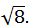(C) 2.                                                                     (D)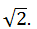(b) the maximum speed of the particle will change by a factor of(A) 4.                                                              (B)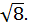(C) 2.                                                              (D)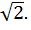(c) the magnitude of the maximum acceleration of the particle will change by a factor of(A) 4.                                                                 (B)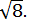(C) 2.                                                                  (D)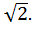(E) 1 (it remains unchanged).

```
5 years agoNavjyot Kalra
654 Points
```							a) The correct option is (D). In simple harmonic motion,1) The time period (T) of vibrations varies inversely as the square root of the force constant (k) of the spring.2) The time period (T) of vibrations varies directly as the square root of the mass (m) of body attached to the string.So the time period of an object of mass m on a spring executes a simple harmonic motion is given by,So, T = 2π √m/kThus in simple harmonic motion, time period of the oscillation is independent of the amplitude.If the mass m of the particle is doubled, then the time period (T ') of the simple harmonic motion would be,T ' = 2π √2m/kSo, T '/ T = (2π √2m/k)/( 2π √m/k) = √2 T ' = √2TFrom the above observation we conclude that, the period of oscillation will change by a factor of √2. Thus option (D) is correct.The correct option is (D).The maximum speed vmax is given by,vmax = xm √k/mHere k is the spring constant, m is the mass of the oscillator and xm is the amplitude of oscillation.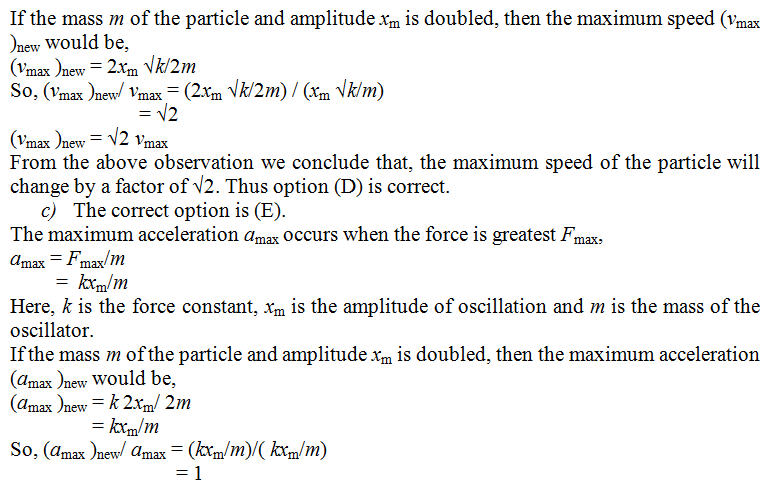(amax )new = amaxFrom the above observation we conclude that, the magnitude of maximum acceleration of the particle will change by a factor of 1. Thus option (E) is correct.
```
5 years ago
Think You Can Provide A Better Answer ?

## Other Related Questions on Wave Motion

View all Questions »### Course Features

• 101 Video Lectures
• Revision Notes
• Previous Year Papers
• Mind Map
• Study Planner
• NCERT Solutions
• Discussion Forum
• Test paper with Video Solution### Course Features

• 110 Video Lectures
• Revision Notes
• Test paper with Video Solution
• Mind Map
• Study Planner
• NCERT Solutions
• Discussion Forum
• Previous Year Exam Questions